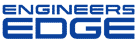Beam Deflection and Analysis

• This calculator contains numerous “comment boxes” which contain a wide variety of information including explanations of input or output items, equations used, data tables, etc. (Note: presence of a “comment box” is denoted by a “red triangle” in the upper right-hand corner of a cell. Merely move the mouse pointer to the desired cell to view the contents of that particular "comment box".)
• This calculator follows the procedures and guidelines of the AISC 9th Edition Allowable Stress (ASD) Manual (1989).
• In this calculator for members subjected to known loadings consisting of axial load (compression or tension) and/or uniaxial or biaxial bending, both the actual and allowable stress are computed, with the final result being a computed "stress ratio" of actual stress/allowable stress.
• This calculator utilizes an "Allowable Stress Increase Factor" (ASIF) which is a multiplier of any of the calculated allowable stresses Fa, Fbx, and Fby and also the Euler column buckling stresses F'ex and F'ey. It is used and appears ONLY in the stress ratio calculation. Typically a value of 1.0 may be used. However, a value of 1.333 may be used for load combinations which include wind or seismic loads.
• If an axially loaded compression member has a value of the maximum slenderness ratio K*L*12/r >200, then a message will appear. However, this calculator DOES NOT consider or deem a particular member as"inadequate" based on the slenderness ratio of 200 being exceeded.
• For the case of combined axial compression with bending, if the calculated value of fa >=F'e (which is not allowed) then a warning (error!) message will appear.
• When the values of either 'Lx', 'Ly', or 'Lb' are input = 0' (or actually <= 1.0'), this calculator will use a value = 1.0'. 11. When a stiffened element (web) of a member subjected to axial compression is classified as a "slender" element (exceeding non-compact limits) based on local buckling criteria, then the calculator complies with AISC Appendix B.
• The values of 'Cb', 'Cmx', 'Cmy', 'Kx, and 'Ky' may be calculated (if applicable) by accessing the additional input data to the right of the main page in each of the calculation worksheets. Then, these calculated values can be input under the member design parameters on the main page. (Note: there are equations which very closely approximate the solutions for 'Kx' and 'Ky' obtained using the AISC Code Alignment Charts.)
• This calculator does not calculate or check shear or deflection in member
• This calculator does not consider torsion on member.
• This calculator does not consider deduction for holes in members subjected to tension.﻿Membership Register | LoginHomeEngineering Book StoreEngineering ForumExcel App. DownloadsOnline Books & ManualsEngineering NewsEngineering VideosEngineering CalculatorsEngineering ToolboxGD&T Training Geometric Dimensioning TolerancingDFM DFA TrainingTraining Online EngineeringAdvertising CenterCopyright Notice